Homework Help Question & Answers

# Calculate the pH of a buffer solution containing 0.100 M CH3COOH and 0.100 M CH3COONa ;...

Calculate the pH of a buffer solution containing 0.100 M CH3COOH and 0.100 M CH3COONa ; Ka of CH3COOH = 1.8 x 10-5

#### Homework Answers

Answer #1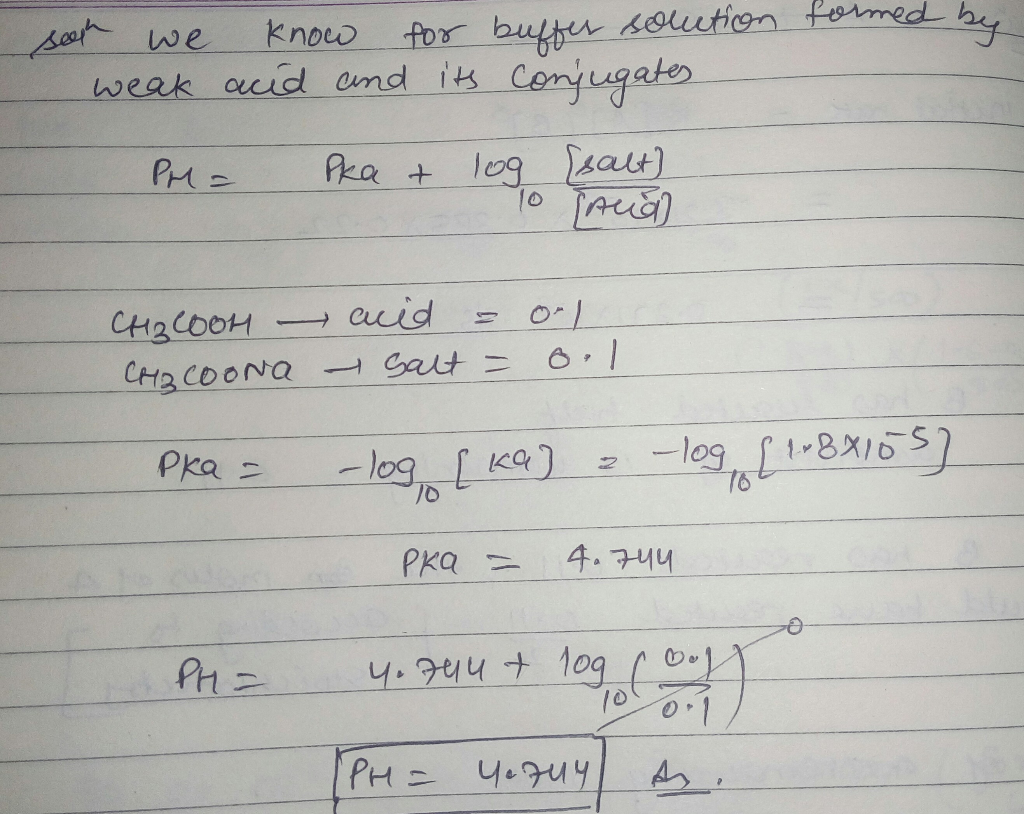Know the answer?
Your Answer:

#### Post as a guest

Your Name:

What's your source?

#### Earn Coin

Coins can be redeemed for fabulous gifts.

Not the answer you're looking for? Ask your own homework help question. Our experts will answer your question WITHIN MINUTES for Free.
Similar Homework Help Questions
• ### Which of the following pH values are within the buffer range for a buffer containing 0.5 M CH3COOH and 0.5 M CH3COONa? T...

Which of the following pH values are within the buffer range for a buffer containing 0.5 M CH3COOH and 0.5 M CH3COONa? The Ka for CH3COO– is 1.8×10–5. Select any answers that apply. Multiple tries are permitted; however, 25% (1/4) point will be deducted for each incorrect response. Select one or more: 2.76 5.09 0.57 3.81 8.11

• ### 14) 14) Calculate the mole ratio of CH3COONa to CH3COOH required to make a buffer with...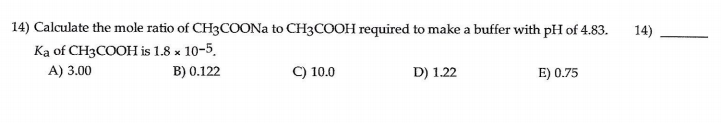14) 14) Calculate the mole ratio of CH3COONa to CH3COOH required to make a buffer with pH of 4.83. Ka of CH3COOH is 1.8 x 10-5. A) 3.00 B) 0.122 C) 10.0 D) 1.22 E) 0.75

• ### What is the pH of a buffer that consists of 0.45 M CH3COOH and 0.35 M...

What is the pH of a buffer that consists of 0.45 M CH3COOH and 0.35 M CH3COONa? Ka = 1.8 x 10-5 So the answer is 4.64. However, I was under the impression that it is Ka * (base/acid). So wouldn't that be 1.8 x 10^-5 * (.35/.45)? Isn't the CH3COONa the base? Someone please explain. Thank you.

• ### 5. Please calculate the pH of a solution containing 0.40 M CH,COOH and 0.70 M CH3COONa...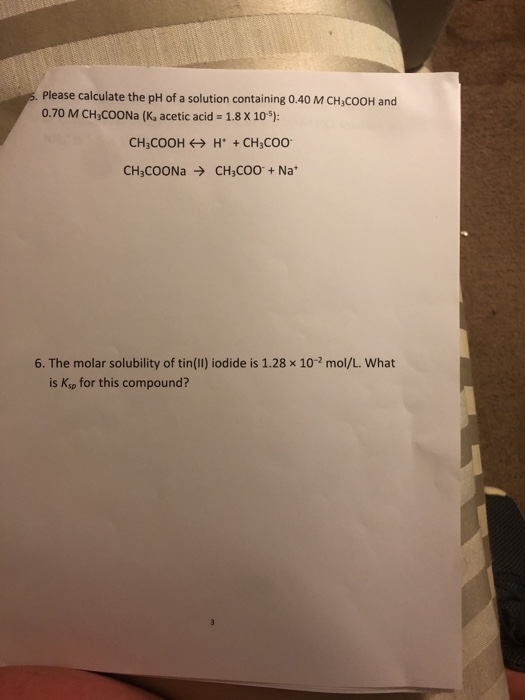5. Please calculate the pH of a solution containing 0.40 M CH,COOH and 0.70 M CH3COONa (K, acetic acid = 1.8 X 10"): CH3COOH + H+ + CH3COO CH3COONa → CH3COO + Nat 6. The molar solubility of tin(ll) iodide is 1.28 x 10-2 mol/L. What is Ksp for this compound?

• ### calculate the ph of a buffer solution that contains 1.5 M acetic acid (CH3COOH) and 0.3...

calculate the ph of a buffer solution that contains 1.5 M acetic acid (CH3COOH) and 0.3 M sodium acetate (CH3COONa) [Ka=1.8x10-5 for acetic acid]

• ### QUESTION 8 Calculate the pH of a solution that is 0.384 M CH3COOH and 0.374 M...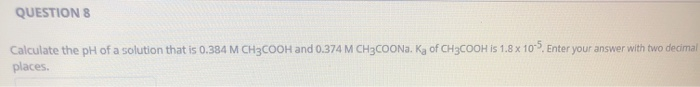QUESTION 8 Calculate the pH of a solution that is 0.384 M CH3COOH and 0.374 M CH3COONa. Ka of CH3COOH is 1.8 x 10". Enter your answer with two decimal places.

• ### Calculate the pH of 1.0L of the buffer 2.00M CH3COONa/2.00M CH3COOH before and after the addition...

Calculate the pH of 1.0L of the buffer 2.00M CH3COONa/2.00M CH3COOH before and after the addition of 0.090 mol NaOH. The Ka of CH3COOH is 1.8x10^-5

• ### 7. Calculate the mass of sodium acetate (CH3COONa) that must be added to 1.00 L 0.450 M acetic acid (CH3COOH), Ka =...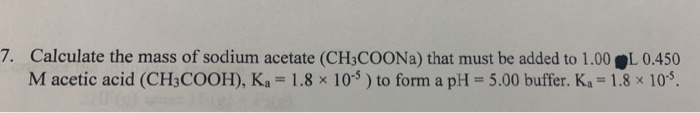7. Calculate the mass of sodium acetate (CH3COONa) that must be added to 1.00 L 0.450 M acetic acid (CH3COOH), Ka = 1.8 x 10 ) to form a pH = 5.00 buffer. Ka = 1.8 x 109.

• ### What is the pH of a buffer that consists of 0.85 M CH3COOH and 0.35 M...

What is the pH of a buffer that consists of 0.85 M CH3COOH and 0.35 M CH3COONa? Ka = 1.8 × 10–5 Select one: A. 4.64 B. 4.31 C. 5.52 D. 5.00 E. 4.85

• ### Calculate the pH of a solution when 100.0 mL of a 0.100 M solution of acetic...

Calculate the pH of a solution when 100.0 mL of a 0.100 M solution of acetic acid (CH3COOH), which has a Ka value of 1.8 × 10–5, is titrated with 110 mLs of a 0.10 M NaOH solution.